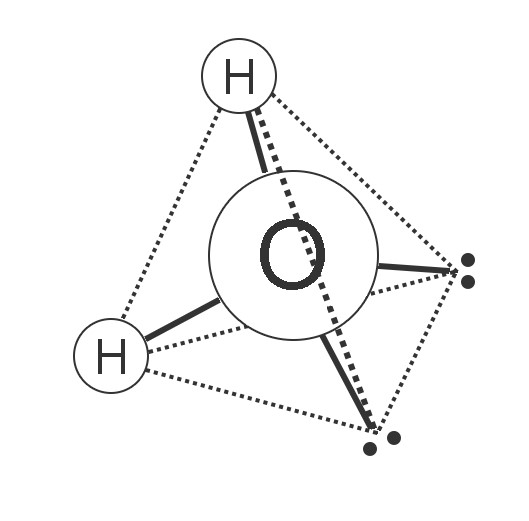# Why does ice float?

and...

## Does ice weigh more than water?

No.

I’m assuming the question refers to equal volumes of each, so it is really asking which is denser, water or ice.

We can see that ice floats in water. So it must be lighter than the water. To understand why that might be, we need to look closely at a molecule of water.

A water molecule forms a lopsided tetrahedron. The two hydrogens are at two corners of the pyramid, and the other two corners have pair of electrons. The electrons repel one another, and that causes the tetrahedron to be somewhat lopsided, so the angle between the two hydrogens is 104.5° instead of the 109.5° that a perfect tetrahedron would have.A water molecule is a tetrahedron

The hydrogens are slightly positive, and the electron pairs are slightly negative, so if it was cold enough the molecules would stick together with a hydrogen of one molecule attracting the electron pair of another. This arrangement makes a six sided crystal, and the molecules are about 9% farther apart than they would be if they were just randomly jumbled together, as they are in liquid water.

This is why an iceberg has 91% of its volume underwater, and only 9% above the water. You can see this when you look at an ice cube floating in a glass of lemonade.

Water generally has air dissolved in it. As the water molecules form nice crystals of ice, the air comes out of solution. This forms tiny bubbles of air in the ice, which is why most ice cubes look white instead of clear. The bubbles make the ice float even higher than it would without them.

You can make ice without bubbles. Boil some water, so that most of the air leaves the water. Then transfer the water to the ice cube tray, and freeze it. If there are still some bubbles in the ice, you can thaw the ice again, letting the bubbles escape, and refreeze it. The result will be crystal clear ice cubes.